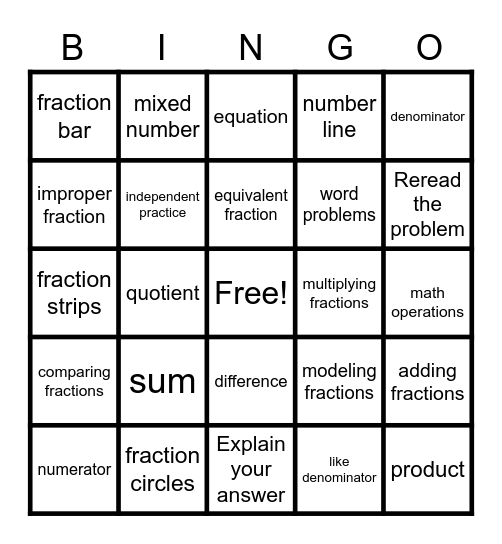# Multiplying Fractions 5/6/20This bingo card has a free space and 24 words: sum, fraction circles, equivalent fraction, numerator, denominator, fraction bar, improper fraction, mixed number, multiplying fractions, difference, word problems, fraction strips, modeling fractions, adding fractions, independent practice, like denominator, math operations, equation, Reread the problem, quotient, product, comparing fractions, number line and Explain your answer.

## Play Online

Share this URL with your players:For more control of your online game, create a clone of this card first.

## Probabilities

With players vying for a you'll have to call about __ items before someone wins. There's a __% chance that a lucky player would win after calling __ items.

Tip: If you want your game to last longer (on average), add more unique words/images to it.